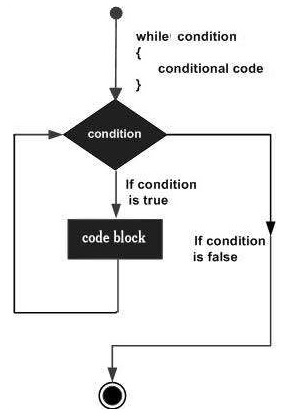# Swift - While Loop

A while loop statement in Swift 4 programming language repeatedly executes a target statement as long as a given condition is true.

## Syntax

The syntax of a while loop in Swift 4 programming language is −

```while condition {
statement(s)
}
```

Here statement(s) may be a single statement or a block of statements. The condition may be any expression. The loop iterates while the condition is true. When the condition becomes false, the program control passes to the line immediately following the loop.

The number 0, the strings '0' and "", the empty list(), and undef are all false in a Boolean context and all other values are true. Negation of a true value by ! or not returns a special false value.

## Flow DiagramThe key point of a while loop is that the loop might not ever run. When the condition is tested and the result is false, the loop body will be skipped and the first statement after the while loop will be executed.

## Example

```var index = 10

while index < 20 {
print( "Value of index is \(index)")
index = index + 1
}
```

Here we are using comparison operator < to compare the value of the variable index against 20. While the value of index is less than 20, the while loop continues executing a block of code next to it and as soon as the value of index becomes equal to 20, it comes out. When executed, the above code produces the following result −

```Value of index is 10
Value of index is 11
Value of index is 12
Value of index is 13
Value of index is 14
Value of index is 15
Value of index is 16
Value of index is 17
Value of index is 18
Value of index is 19
```
swift_loops.htm